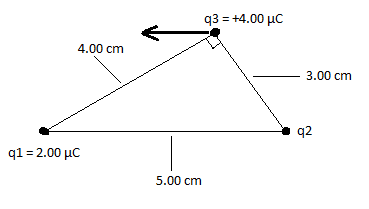# How to find the magnitude of a point charge given 2 others?

shqiptargirl

## Homework Statement

Three charges are placed as shown in the figure below. The magnitude of q1 is 2.00 µC, but its sign is not known. The charge and sign of q2 is not known. We do know that q3 is +4.00 µC and the net force on q3 is entirely in the negative x-direction.a.) Deduce the signs of charges q1 and q2.
b.) Figure out the magnitude of q2

## Homework Equations

Coulomb's law:

F = (K)*(q1)(q2)/d^2

## The Attempt at a Solution

I know how to determine the charges of q1 and q2. Since the y component of the net force vector of q3 equals 0, then q1 must be negative and q2 must be positive.

Besides that, I have no idea on how to start figuring out the magnitude of q2

## Answers and Replies

Homework Helper
Gold Member
Since the y component of the net force vector of q3 equals 0, then q1 must be negative and q2 must be positive.
Well, it could have been the other way around, but then the net force would have been in the +ve x direction.
Your observation about net zero in the y direction tells you more than you've used. What is the y direction force from q1 on q3?

shqiptargirl
Well, it could have been the other way around, but then the net force would have been in the +ve x direction.
Your observation about net zero in the y direction tells you more than you've used. What is the y direction force from q1 on q3?

The y direction force from q1 on q3 is 23.15 N. So The y direction force from q2 from q3 must be -23.15 N ? The problem is I don't know how to use this information to solve for the magnitude of q2.

Also, what does this mean, "+ve x direction" ? I didn't understand your comment on how it could've been the other way around (sorry) :(

Homework Helper
Gold Member
The y direction force from q1 on q3 is 23.15 N
I don't know k off the top of my head so I can't quickly check that. For the purposes of the question, the value of k is immaterial, so we can pretend it's 1 whatever, consistent with expressing distances in cm and charges in µC.
The y direction force from q2 from q3 must be -23.15 N ?
Yes, that would follow. You know the magnitude of q3, and the position of q2 in relation to it, so you can write an equation for that force as a function of q2's magnitude.
what does this mean, "+ve x direction"
Sorry, +ve means positive, -ve means negative. I thought these were very standard.
I didn't understand your comment on how it could've been the other way around
You could set q1 positive and q2 negative and still arrange that there's not net Y field at q3. So you need to use the fact that the X field at q3 is to the left to determine which is positive and which is negative.

shqiptargirl
You said this... "Yes, that would follow. You know the magnitude of q3, and the position of q2 in relation to it, so you can write an equation for that force as a function of q2's magnitude."

I don't understand this part. I am lost on how to do this.
And k btw is about 9 *10 ^9, atleast that is what I have been using.

Homework Helper
Gold Member
Hm, but if you flipped them around, wouldn't the arrow be pointing in the opposite direction? If you look at the diagram the arrow is pointing to the left. If you made q1 positive and q2 negative, the arrow would be pointing to towards the right, no?
Yes, that's exactly what I'm saying. In your original post you wrote
Since the y component of the net force vector of q3 equals 0, then q1 must be negative and q2 must be positive.
as though the zero y component was enough to determine that q1 must be negative and q2 must be positive. That observation only tells you they are of opposite sign. To deduce that it's q1 that's negative you also need to use the direction in which the arrow points.
You probably worked all that through when you made the post, but misrepresented your own logic.

It really is a very small point, and I'd rather get on with finding q2.

shqiptargirl
I know, I am sorry. I didn't see the last part of your reply because of the computer screen I am using.